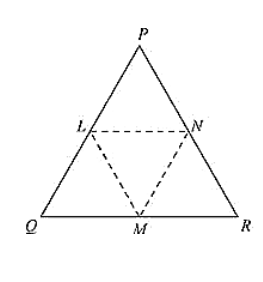# In a Δ PQR. IF PQ = QR and L, M and N are the mid-points of the sides PQ,

Question:

In a Δ PQR. IF PQ = QR and L, M and N are the mid-points of the sides PQ, QR and RP respectively. Prove that LN = MN.

Solution:

Given that in ΔPQR, PQ = QR and L, M and N are the mid-points of the sides PQ, QR and RP respectively

We have to prove LN = MN.

Join L and M, M and N, N and L

We have PL = LQ, QM = MR and RN = NP

[Since, L, M and N are mid-points of Pp. QR and RP respectively]

And also PQ = QR

PL = LQ = QM = MR = PQ/2 = QR/2 ... (i) Using mid-point theorem,

We have

MN ∥ PQ and MN = PQ/2

MN = PL = LQ  ... (ii)

Similarly, we have

LN ∥ QR and LN = (1/2)QR

LN = QM = MR ... (iii)

From equation (i), (ii) and (iii), we have

PL = LQ = QM = MR = MN = LN

LN = MN